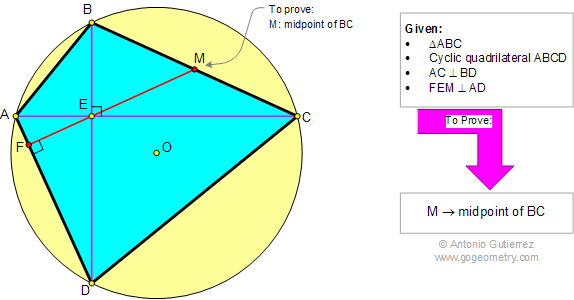###### Online Geometry Problem 871: Brahmagupta's Theorem, Cyclic Quadrilateral, Perpendicular Diagonals, Midpoint. Level: High School, College, Mathematics Education

Brahmagupta's theorem states that if a cyclic quadrilateral ABCD has perpendicular diagonals, then the perpendicular to a side from the point of intersection of the diagonals always bisects the opposite side.Brahmagupta (598–668) was an Indian mathematician and astronomer who discovered a neat formula for the area of a cyclic quadrilateral.